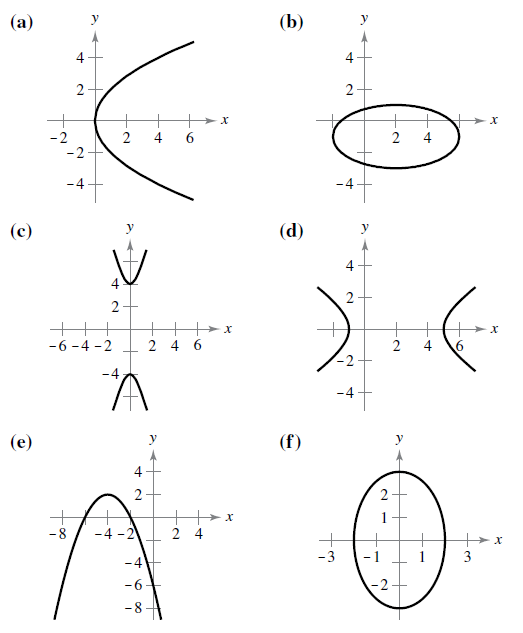Chapter 10.1, Problem 1E

Chapter
Section
Textbook Problem

# Matching In Exercises 5-10, match the equation with its graph. [The graphs are labeled (a), (b), (c), (d), (e). and (f).]y 2 = 4 x

To determine
The correct match of the provided equation y2=4x with its correct graph.

Explanation

Given: The equation y2=4x and the graph of equations are,

Calculation: Consider the equation y2=4x. Since the provided equation is of the type y2=4ax which is the equation of the parabola with vertex at the origin and the length of latus rectum 4a and the focus is at. For the given equation latus rectum is 41, and the focus is at (1,0). From the equation, it is observed that the parabola whose vertex is at the origin and of the type y2=4x is an option (a). Hence the appropriate graph of the equation y2=4x is,

### Still sussing out bartleby?

Check out a sample textbook solution.

See a sample solution

#### The Solution to Your Study Problems

Bartleby provides explanations to thousands of textbook problems written by our experts, many with advanced degrees!

Get Started

#### Evaluate Evaluate the expression. 12. 23 32

Precalculus: Mathematics for Calculus (Standalone Book)

#### Solve the equations in Exercises 126. (x2+1)x+1(x+1)3=0

Finite Mathematics and Applied Calculus (MindTap Course List)

#### Solve for if 0360. 3sincos=1

Trigonometry (MindTap Course List)